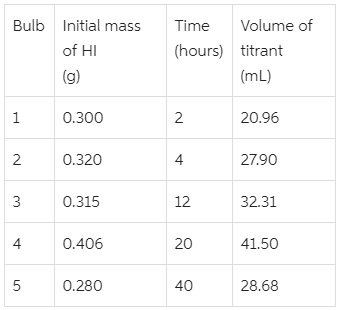# Problem: The decomposition of HI(g) is represented by the equation2HI(g) ⇌ H2(g) + I2(g)The following experiment was devised to determine the equilibrium constant of the reaction. HI (g) is introduced into five identical 400-cm3 glass bulbs, and the five bulbs are maintained at 623 K. The amount of I2 produced over time is measured by opening each bulb and titrating the contents with 0.0150 M Na2S2O3 (aq). The reaction of I2 with the titrant isI2 + 2Na2S2O3 ⇌ Na2S4O6 + 2NaIData for the experiment are provided in this table.What is the value of Kc for the decomposition of HI at 623 K?

###### FREE Expert Solution

We’re asked to determine the equilibrium constant Kc for the decomposition of HI at 623 K, based on the titration data.

In equilibrium, the amount of I2 produced is the same amount that was titrated by Na2S2O3.

Titration reaction occurs in a 1:2 mole ratio, where 1 mole of I2 reacts with 2 moles of Na2S2O3.

This way, we can determine the amount of Iin the decomposition reaction.

To determine Kc, we do the following steps:

Step 1: Calculate the moles of I2 in equilibrium, from the volume of titrant used.

95% (255 ratings)###### Problem Details

The decomposition of HI(g) is represented by the equation

2HI(g) ⇌ H2(g) + I2(g)

The following experiment was devised to determine the equilibrium constant of the reaction. HI (g) is introduced into five identical 400-cm3 glass bulbs, and the five bulbs are maintained at 623 K. The amount of I2 produced over time is measured by opening each bulb and titrating the contents with 0.0150 M Na2S2O3 (aq). The reaction of I2 with the titrant is

I2 + 2Na2S2O3 ⇌ Na2S4O6 + 2NaI

Data for the experiment are provided in this table.What is the value of Kc for the decomposition of HI at 623 K?